Courses

# Test: Patterns

## 10 Questions MCQ Test Mathematics (Maths) for Class 1 | Test: Patterns

Description
This mock test of Test: Patterns for Class 1 helps you for every Class 1 entrance exam. This contains 10 Multiple Choice Questions for Class 1 Test: Patterns (mcq) to study with solutions a complete question bank. The solved questions answers in this Test: Patterns quiz give you a good mix of easy questions and tough questions. Class 1 students definitely take this Test: Patterns exercise for a better result in the exam. You can find other Test: Patterns extra questions, long questions & short questions for Class 1 on EduRev as well by searching above.
QUESTION: 1

Solution:
QUESTION: 2

### Choose the figure that is different from the following group.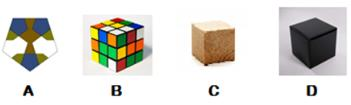Solution:

A is pentagon and all other are Cube.

QUESTION: 3

### What is the shape of the mirror?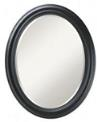Solution:
QUESTION: 4

How many circles are there in pattern given?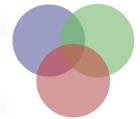Solution:
QUESTION: 5

How many green triangles are there in the given pattern?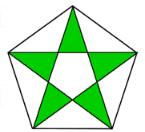Solution:
QUESTION: 6

How many circles are given in the picture?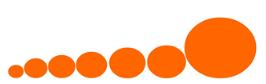Solution:
QUESTION: 7

Identify the number of yellow squares in the picture.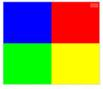Solution:

Only 1 YELLOW square is present.

QUESTION: 8

Identify the odd one out from the following options.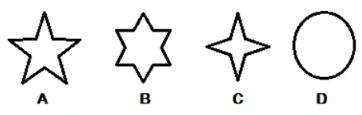Solution:
QUESTION: 9

What are the shapes in the pattern given here?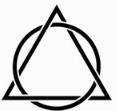Solution:
QUESTION: 10

A  _________ is divided into two halves. Fill in the blanks from the options given below.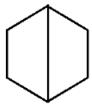Solution:

Hexagon